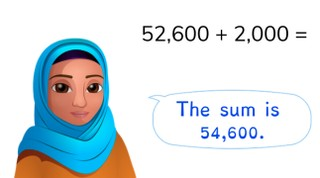Addition to 100,000 with simple numbers

# Addition to 100,000 with simple numbers

Students learn to add to 100,000 with simple numbers.8,000 schools use Gynzy92,000 teachers use Gynzy1,600,000 students use Gynzy

## General

Students learn to add to 100,000 with simple numbers.

## Standards

CCSS.Math.Content.4.NBT.B.4

## Learning objective

Students will be able to add to 100,000 with simple numbers.

## Introduction

Have the students determine which number belongs with which fruit by solving the math problems.

## Instruction

Show the sum 72,300 + 21,000. Explain that you start by adding up the ten thousands numbers. 70,000 + 20,000 = 90,000. Then you add the thousands numbers together. 2,000 + 1,000 = 3,000. Next the hundreds numbers. Now add all the answers together. 90,0000 + 3,000 + 300 = 93,300. Have the students solve the next few problems on their own. Show the story problem. Explain that you first take the problem out of the story. Then you add the ten thousands numbers together.

Check whether the students can add to 100,000 with simple numbers with the following question:
- How can you add to 100,000 in steps with simple numbers?

## Quiz

The students first practice two assignments involving addition to 100,000. The third assignment is a story problem.

## Closing

Discuss with the students the importance of being able to add to 100,000 with simple numbers. As a closing activity you can have the students choose a number, spin the spinner and solve the math problem.

## Teaching tips

Students that have difficulty with addition to 100,000 with simple numbers can first practice adding numbers to 1,000 and 10,000.

### The online teaching platform for interactive whiteboards and displays in schools

• Save time building lessons

• Manage the classroom more efficiently

• Increase student engagement